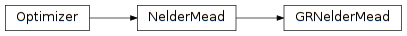# 4.7. pyopus.optimizer.grnm — Grid-restrained Nelder-Mead simplex optimizer¶Unconstrained grid-restrained Nelder-Mead simplex optimizer (PyOPUS subsystem name: GRNMOPT)

A provably convergent version of the Nelder-Mead simplex algorithm. The algorithm performs unconstrained optimization. Convergence is achieved by restraining the simplex points to a gradually refined grid and by keeping the simplex internal angles away from 0.

The algorithm was published in

 [grnm] Bűrmen Á., Puhan J., Tuma T.: Grid Restrained Nelder-Mead Algorithm. Computational Optimization and Applications, vol. 34, pp. 359-375, 2006.

There is an error in Algorithm 2, step 5. The correct step 5 is: If \$f^{pe}<min(f^{pe}, f^1, f^2, ..., f^{n+1})\$ replace \$x^i\$ with \$x^{pe}\$ where \$x^{i}\$ denotes the point for which \$f(x^i)\$ is the lowest of all points.

class pyopus.optimizer.grnm.GRNelderMead(function, debug=0, fstop=None, maxiter=None, reflect=1.0, expand=1.2, outerContract=0.5, innerContract=-0.5, shrink=0.25, reltol=1e-15, ftol=1e-15, xtol=1e-09, simplex=None, lam=2.0, Lam=4503599627370496.0, psi=1e-06, tau_r=2.220446049250313e-16, tau_a=1e-100, originalGrid=False, gridRestrainInitial=False)

Unconstrained grid-restrained Nelder-Mead optimizer class

Default values of the expansion (1.2) and shrink (0.25) coefficients are different from the original Nelder-Mead values. Different are also the values of relative tolerance (1e-16), and absolute function (1e-16) and side length size (1e-9) tolerance.

lam and Lam are the lower and upper bound on the simplex side length with respect to the grid. The shape (side length determinant) is bounded with respect to the grid density by psi.

The grid density has a continuity bound due to the finite precision of floating point numbers. Therefore the grid begins to behave as continuous when its density falls below the relative(tau_r) and absolute (tau_a) bound with respect to the grid origin.

If originalGrid is True the initial grid has the same density in all directions (as in the paper). If False the initial grid density adapts to the bounding box shape.

If gridRestrainInitial is True the points of the initial simplex are restrained to the grid.

buildGrid(density=10.0)

Generates the intial grid density for the algorithm. The grid is determined relative to the bounding box of initial simplex sides. density specifies the number of points in every grid direction that covers the corresponding side of the bounding box.

If any side of the bounding box has zero length, the mean of all side lengths divided by density is used as grid density in the corresponding direction.

Returns the 1-dimensional array of length ndim holding the grid densities.

check()

Checks the optimization algorithm’s settings and raises an exception if something is wrong.

gridRestrain(x)

Returns the point on the grid that is closest to x.

reset(x0)

Puts the optimizer in its initial state and sets the initial point to be the 1-dimensional array or list x0. The length of the array becomes the dimension of the optimization problem (ndim member).

The initial simplex is built around x0 by calling the buildSimplex() method with default values for the rel and abs arguments.

If x0 is a 2-dimensional array or list of size (ndim*+1) times *ndim it specifies the initial simplex.

A corresponding grid is created by calling the buildGrid() method.

The initial value of the natural logarithm of the simplex side vectors determinant is calculated and stored.

reshape(v=None, Q=None, R=None)

Reshapes simpex side vectors given by rows of v into orthogonal sides with their bounding box bounded in length by lam and Lam with respect to the grid density. If v is None it assumes that it is a product of matrices Q and R.

Returns a tuple (vnew, l) where vnew holds the reshaped simplex sides and l is the 1-dimensional array of reshaped side lengths.

run()

Runs the optimization algorithm.

sortedSideVectors()

Returns a tuple (vsorted, lsorted) where vsorted is an array holding the simplex side vectors sorted by their length with longest side first. The first index of the 2-dimensional array is the side vector index while the second one is the component index. lsorted is a 1-dimensional array of corresponding simplex side lengths.

#### Previous topic

4.6. pyopus.optimizer.nm — Unconstrained Melder-Mead simplex optimizer

#### Next topic

4.8. pyopus.optimizer.sanm — Unconstrained successive approximation Nelder-Mead simplex optimizer